# Double six-six

What is the probability, that a player in the games of ludo will throw a double 6 twice .

p =  0.0008

### Step-by-step explanation: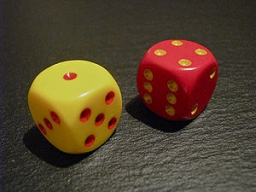Did you find an error or inaccuracy? Feel free to write us. Thank you!Tips to related online calculators
Would you like to compute count of combinations?

## Related math problems and questions:

• ThrowWe throw 2 times with 2 dices. What is the probability that the first roll will fall more than sum of 9 and the second throw have sum 3 or does not have the sum 4?
• How many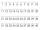How many double-digit numbers greater than 30 we can create from digits 0, 1, 2, 3, 4, 5? Numbers cannot be repeated in a two-digit number.
• Dd 2-digit numbersFind all odd 2-digit natural numbers compiled from digits 1; 3; 4; 6; 8 if the digits are not repeated.
• One threeWe throw two dice. What is the probability that max one three falls?
• Three diceThe player throwing the three dice asked G. Galilei the question: "Should I bet on the sum of 11 or the sum of 12?" What did Galilei answer him? Hint: write down all three triples of numbers that can be thrown and: have a total of 11 have a total of 12 an
• Telephone numbers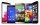How many 7-digit telephone numbers can we put together so that each number consists of different digits?
• The dice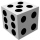Find the probability that a number divisible by two or three will fall when the dice are rolled.
• DiceWe throw five times the dice. What is the probability that six fits exactly twice?
• DiceWe throw 10 times a playing dice. What is the probability that the six will fall exactly 4 times?
• Games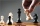Jack and Paul decided to play chess against each other. They bet ten pesos on each game they played. Jack won three bets and Paul won fifty pesos. How many games did they play?
• Seven-segmet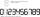Lenka is amused that he punched a calculator (seven-segment display) numbers and used only digits 2 to 9. Some numbers have the property that their image in the axial or central symmetry was again give some number. Determine the maximum number of three-di
• Two diceWe roll two dice. What is the probability that the sum of the falling numbers is greater than 3?
• We rollWe roll two dice A. - what is the probability that the sum of the falling numbers is at most 4 B. - is at least 10 C. - is divisible by 5?
• Right key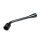The hostel has 4 rooms. The keys to each room are not numbered. Each of the four guests took one key. What is the probability that everyone took the right key?
• Chess competition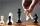4 chess players took part in the competition. How many tournaments have taken place if every chess player has fought everyone once?
• What fraction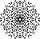What fraction of number 1 to 30 is prime?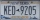A license plate has 3 letters followed by 4 numbers. Repeats are not allowed for the letters, but they are for the numbers. If they are issued at random, what is the probability that the 3 letters are in alphabetical order and the 3 numbers are consecutiv